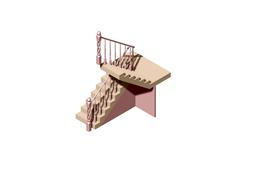# Old Fashioned style staircaseLearn BricsCAD with practical examples. This week, learn how to use advanced modeling techniques to make a set of stairs in CAD.
Commands covered:

DIV(DIVIDE)
AR(ARRAY)/Path
AR(ARRAY)/Polar
SWEEP
REV(REVOLVE)
EXT(EXTRUDE)/Both sides
3P(3DPOLY)
IAT(IMAGEATTACH)

1. ### Step 1: The steps

To create the steps themselves you have two options:

Option 1

1. Start with a box (first step) measured to the correct dimensions.
2. For this example I used: 800 x 160 x 210.3. Use AR to repeat.
4. Array values: Col 7, distance - 210 (width of steps), Row -2, distance - 800 (length of steps).
5. Then EXPLODE the array.
6. Add a rectangle to the end for the middle step.7. Extrude the blocks to the correct height using Push/Pull.Option 2

1. Draw a line to the desired height on the steps.
2. Then use DIV to split the line into segments.
3. In this example I used height - 2240 and divde - 14.Tip: Enter Settings - Drafting - Entity creation - Point display mode, to change how the points are displayed on screen.4. Now extrude each step, using Node Snap to get the height of each step.2. ### Step 2: The Balusters

The next phase was making the balusters. I used a photograph of a baluster, traced it, and revolved. Then I aligned the balusters along the stairs.

To do this:

1. First import an image into BricsCAD use the Attach Raster Image (IAT) command.
2. Then, trace one side using SPLINE
3. Next draw a line down the center.4. Finally, use REV - 360°. Use your center line as the axis of rotation.5. Use 3P to draw a line between the end point of the top and middle step.
6. Or see last week's post for drawing on custom user coordinate systems.7. Then, with a little clever geometry and Node Snap, use MOVE to reposition the line to the correct starting point on the first step.
8. Align the baluster center middle with the endpoint of the 3D polyline.
9. Then use ARRAYPATH to position the balusters up the staircase. Read more about Array Path.
10. The settings I used were: Mode - divide, Items - 8.I then repeated for the second half of the staircase.3. ### Step 3: The Newel

To make the newel I used a similar technique to model the balusters. I then extruded rectangles to remove the excess material, chamfered, added columns and applied a twist.To do this:

1. Create your top and base parts in the same way as the balusters.
2. Next use EXT Subtract, Both sides.
3. Apply a chamfer to the hard edges.
4. For this example I used a radius value of 20.
5. Then draw in the columns. Use ARRAYPOLAR to repeat the object 4 times.
6. Use UNI to create a solid object.
7. Finally, apply TWIST. Use the centerline as your axis. Place the start and end points of twisting axis between the rectangular portions to prevent the rectangular portions becoming twisted.4. ### Step 4: Finishing UpI removed the excess material by drawing the cutting line on to the steps and added wall details. Then I imported the newel and extended the handrail.